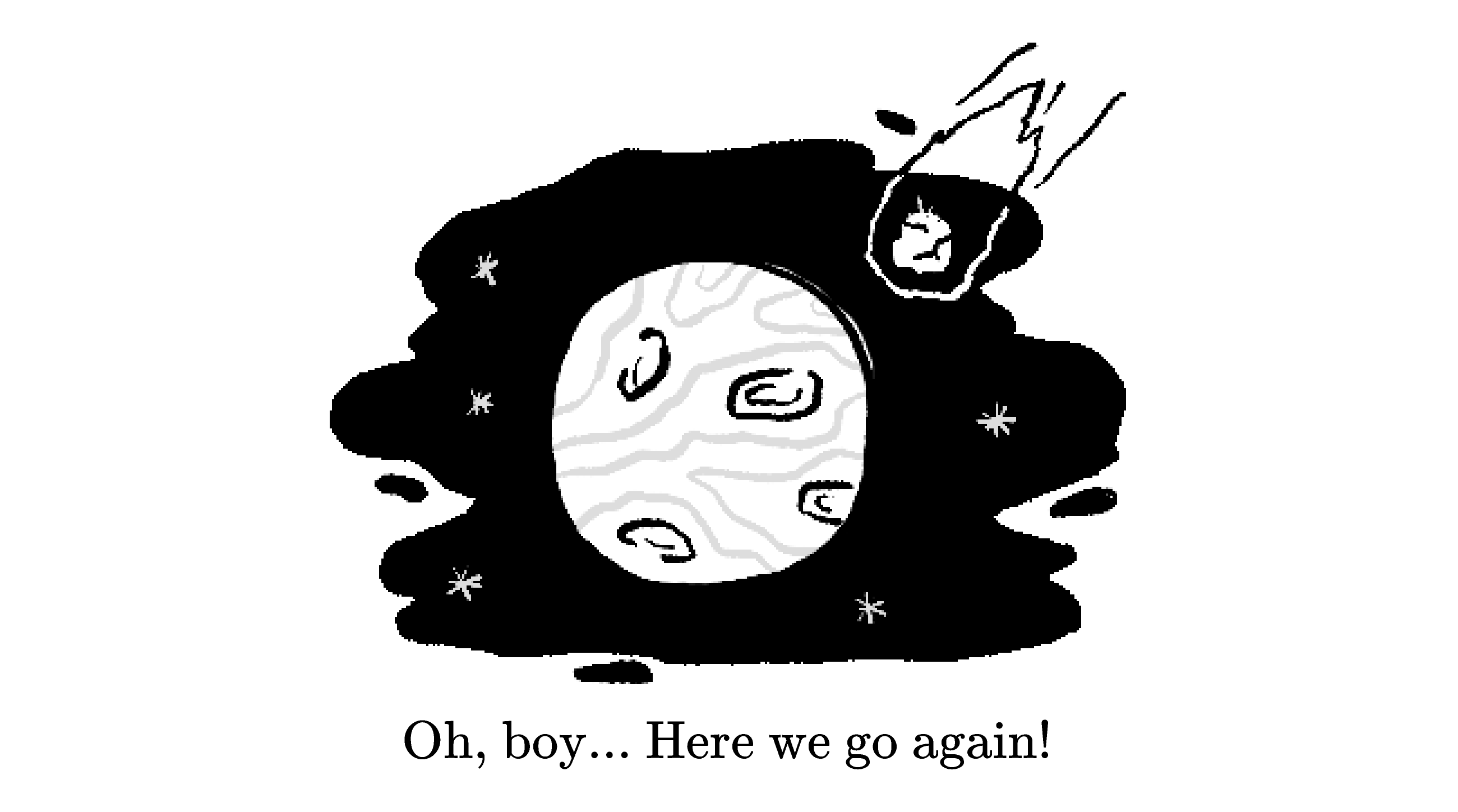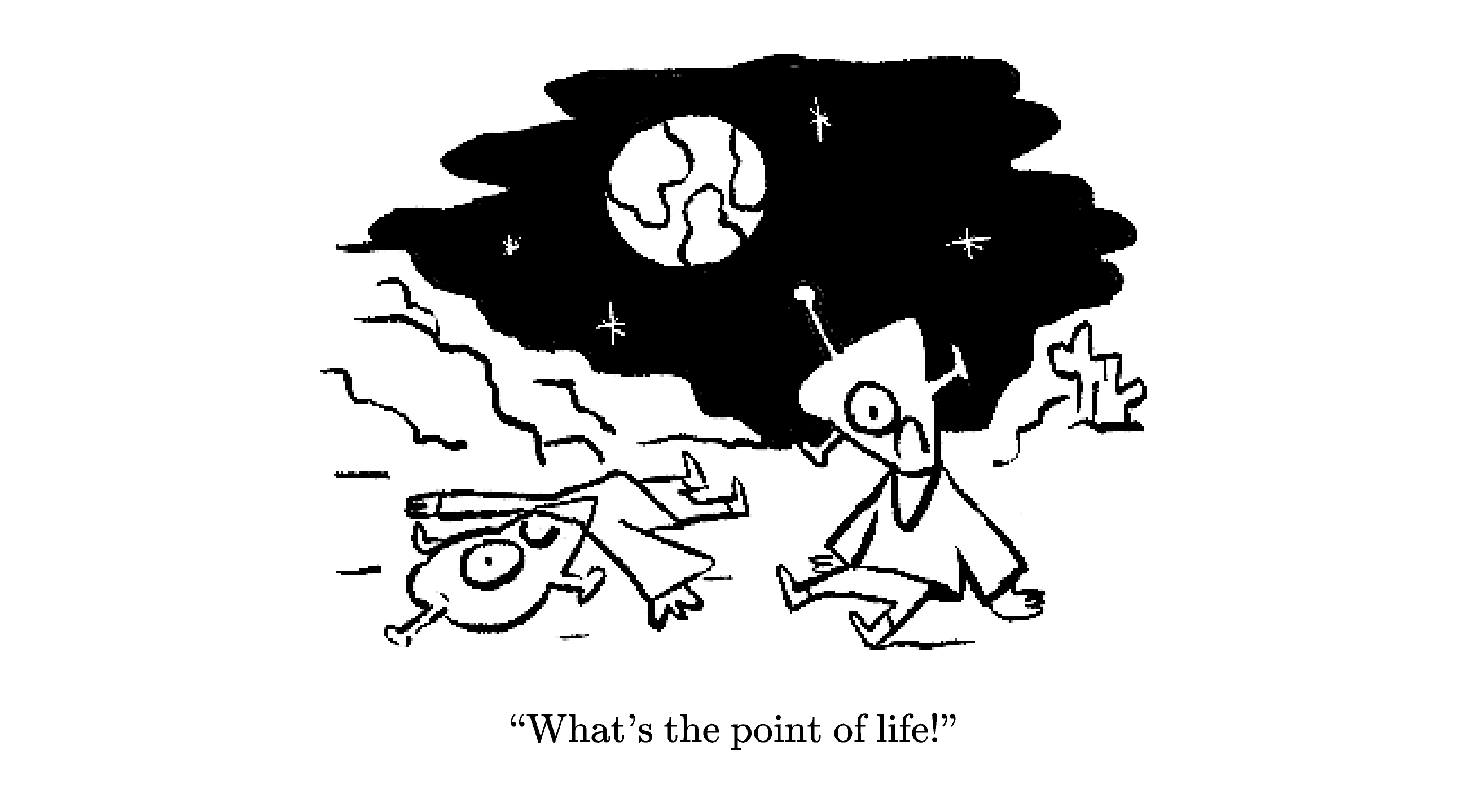### Statistics I

The last quiz outlined the basics of hypothesis testing, which is a pillar of modern statistics.

In hypothesis testing, an assumption is either ruled probably true or probably wrong depending on data gathered from experimentation.

Rupert, the skeptical magician in the last quiz, could ask The Oracle as many questions as he liked, but data gathering in reality is usually difficult and costly.

Sampling is one technique for coping with this issue, and this quiz touches on its basics.

But instead of using sampled data to perform yet another hypothesis test, we'll apply it to another very important statistical problem: parameter estimation.

# Blue Mars: Sampling & Estimation

Billions of years will wear down even the best of civilizations. Just ask the Martians!

Oh, the Martians have had their share of rough patches all right. They've been bombarded by asteroids, comets, and even a small planetoid. They lost their oceans and their protection from solar radiation when the magnetic field vanished like a puff of dust in a killer Martian sandstorm.

And the new neighbors.... well, the less said about the third rock from the Sun, the better.Yeah, it hasn't been a smooth ride, but that's not what irks the modern Martian. See, after billions of years, the Martian civilization has accomplished all it can, and this is depressing the populace.

In a few words, planet Mars is blue because there's nothing left to do!

Concerned for their people, Dr. Ziggy Stardust, professor of Martian psychology, sets out to determine how far this Martian malaise has spread.# Blue Mars: Sampling & Estimation

Fortunately for Ziggy, Martians are pretty easy to study, statistically speaking.

In fact, genetically, they're all clones of one another, so the only feature distinguishing them is their current mental state: "sad" and "not sad."

Now, if Ziggy were to pick any fellow denizen of the red planet at random and ask that Martian how they're feeling, they'd respond "sad" with probability $p.$

If there are $N_{\text{sad}}$ sad Martians, $N_{\text{not sad}}$ not sad Martians, and $N$ Martians in total, how is $p$ related to these numbers?

# Blue Mars: Sampling & Estimation

The proportion $p = \frac{N_{\text{sad}}}N$ is an example of a population parameter, a number quantifying some characteristic of the population at large.

Population parameters are highly sought after, but limitations in resources like time, energy, and money practically rule out any attempts to find their true values in real-world scenarios.

We'll elaborate on this in later quizzes, but for the moment put yourself in Ziggy's shoes and imagine reaching out to billions of Martians in order to chat about their current mood.This would take up an astronomical amount of time, so finding the exact value of $p$ is practically impossible, even for a persistent Martian like Ziggy.

# Blue Mars: Sampling & Estimation

Instead of calling up every Martian, Ziggy decides to collect data from a much smaller sample.

A sample is a subset of the overall population.

Because Martians are uniform in nearly every respect except their mood (sad and not sad), Ziggy thinks a randomly selected sample should represent all of Mars.

Ziggy pulls $50$ names at random from the Mars Directory, calls them up, and records their emotional state.

If $15$ of them report being bummed out, what would be Ziggy's estimate for $p?$

# Blue Mars: Sampling & Estimation

In statistics, an estimator is a rule for estimating some feature of the population from data.

An estimator typically has a "hat" like $\hat{p},$ whereas the true population parameters have no hat. For example, for $p$'s estimator we write $\hat{p} = \frac{n_{\text{sad}}}{n_{\text{sad}}+n_{\text{not sad}}}.$ Here, $n_{\text{sad}}$ and $n_{\text{not sad}}$ are the numbers of sad and not sad Martians in Ziggy's sample, respectively.

# Blue Mars: Sampling & Estimation

Ziggy's estimate of $\hat{p} = 0.3 = 30 \ \%$ for $p,$ the true proportion of dispirited Martians, is a bit surprising.

The Tharsis Times, Mars' premiere newspaper, did their own survey of $50$ randomly chosen Martians and estimated that $\hat{p} = 45 \%$ of the planet suffers from Martian malaise.What's the most reasonable explanation for the difference in the two estimates?

Assume both Ziggy and the Tharsis Times followed exactly the same procedure to get their samples.

# Blue Mars: Sampling & Estimation

When we take multiple independent samples from a population to estimate a parameter, we're very likely to see differences in the results.

These differences, called fluctuations, are due to the randomness inherent to sampling.

For example, a flipped fair coin lands either heads up or tails up with probability $\frac{1}{2},$ but if it's flipped $20$ times in a row, there's no guarantee that we'll see precisely $10 \ \tt H$'s and $10 \ \tt T$'s: one string of $20$ flips may have $8 \ \tt H$'s and $12 \ \tt T$'s, and another may have $17 \ \tt H$'s and $3 \ \tt T$'s.

The average fluctuation size is a good indicator of how much we can trust an estimate: for relatively small sizes, different samples will produce roughly equal estimates on average.

We'll see an example of how to estimate the average fluctuation size later in this quiz.

# Blue Mars: Sampling & Estimation

Ziggy now believes the difference between their estimate of $\hat{p} = 0.3$ and the Tharsis Times estimate of $\hat{p} = 0.45$ is due to statistical fluctuations.

Knowing that "the truth is out there," Ziggy sets out to repeat their experiment but very much wants to avoid the mistake made in their first ill-fated attempt.

What can Ziggy do to decrease the fluctuation in their own estimate?

Hint: Think about the completely hypothetical scenario where the sample consists of the entire Martian population.

# Blue Mars: Sampling & Estimation

So, for larger and larger samples, we expect the fluctuations to decrease.

In the hypothetical case where the sample is the entire population, the estimate for any parameter is exact and the fluctuation is $0.$

Statistics deals with populations that cannot be sampled and sample sizes much smaller than the overall population size, so we must learn to cope with fluctuations in our sample estimates.

Later in the course, we'll study problems exactly like Ziggy's in much greater depth. We'll find out that the average fluctuation size is roughly $\frac{\sqrt{n_{s} \hat{p}(1-\hat{p})}}{n_{s} \hat{p}} = \sqrt{\frac{1-\hat{p}}{\hat{p}}} \frac{1}{\sqrt{n_{s}}}.$ Notice that this formula predicts that as the sample size $n_{s}$ goes up, the average fluctuation size goes down!

# Blue Mars: Sampling & Estimation

Ziggy conducts another survey: this time, $240$ out of $600$ Martians polled report despondency.

Ziggy counts on the larger sample size reducing the fluctuation size.

Estimate the size of the fluctuation for this new sample.

# Blue Mars: Sampling & Estimation

Ziggy estimates that somewhere between $35\%$ and $45\%$ of all Martians are down in the dumps.

Always up for a challenge, Ziggy starts concocting ideas to cheer them up: maybe a new hobby, or a cruise to Jupiter or Saturn... but probably not Earth.To summarize, sampling is a statistician's primary tool when faced with a very large population.

Typically, some number characterizing this population, a population parameter, is of interest but also practically inaccessible.

The statistician estimates the population parameter using data collected from a much smaller subset of the population, called a sample.

Such sampling is subject to fluctuations. Increasing the sample size decreases these fluctuations, but practicalities limit how large a data sample can be.

In the next quiz, we cover the single most important theorem in all of statistics: the central limit theorem. There's no exaggeration in saying that statistics would be impossible without it.

# Blue Mars: Sampling & Estimation

×